# Electrical Engineering - Online Test

Q1.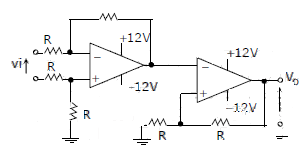The CORRECT transfer characteristic is

Explaination / Solution:

It is a Schmitt trigger and phase shift is zero.

Q2. Given a vector field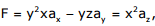the line integral ∫ F.dl evaluated along a segment on the x-axis from x=1 to x=2 is
Explaination / Solution:
No Explaination.

Q3. If R(τ) is the auto correlation function of a real, wide-sense stationary random process, then which of the following is NOT true
Explaination / Solution:
No Explaination.

Q4. A plane wave of wavelength λ is traveling in a direction making an angle 300 with positive x − axis and 900 with positive y − axis. The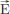field of the plane wave can be represented as (E is constant)
Explaination / Solution: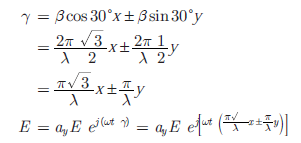Q5. A p n junction has a built-in potential of 0.8 V. The depletion layer width a reverse bias of 1.2 V is 2 μm. For a reverse bias of 7.2 V, the depletion layer width will be
Explaination / Solution: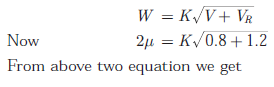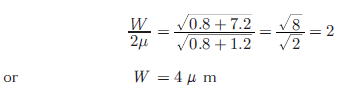Q6.
An open loop system represented by the transfer functionis
Explaination / Solution:

Open loop system stability is depends only on pole locations ⇒ system is stable There is one zero on right half of s-plane so system is non – minimum phase

Q7. With K as a constant, the possible solution for the first order differential equationis
Explaination / Solution:Q8. The fourier series expansionof the periodic signal shown below will contain the following nonzero terms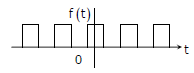Explaination / Solution:

⇒ it satisfies the half wave symmetry, so that it contains only odd harmonics.
⇒ It satisfies the even symmetry. So bn = 0

Q9. A three-phase, salient pole synchronous motor is connected to an infinite bus. Ig is operated at no load a normal excitation. The field excitation of the motor is first reduced to zero and then increased in reverse direction gradually. Then the armature current
Explaination / Solution:
No Explaination.

Q10. Consider the following statement (i) The compensating coil of a low power factor wattmeter compensates the effect of the impedance of the current coil. (ii) The compensating coil of a low power factor wattmeter compensates the effect 0of the impedance of the voltage coil circuit.
Explaination / Solution:
No Explaination.